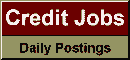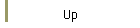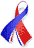DefaultRisk.com the web's biggest credit risk modeling resource.Export citation to:- HTML- Text (plain)- BibTeX- RIS- ReDIF

Multiscale Stochastic Volatility Asymptotics

by Jean-Pierre Fouque of North Carolina State University,
George Papanicolaou of Stanford University,
Ronnie Sircar of Princeton University, and
Knut Sølna of the University of California, Irvine

October 2003

Abstract: In this paper we propose to use a combination of regular and singular perturbations to analyze parabolic PDEs that arise in the context of pricing options when the volatility is a stochastic process that varies on several characteristic time scales. The classical Black-Scholes formula gives the price of call options when the underlying is a geometric Brownian motion with a constant volatility. The underlying might be the price of a stock or an index, say, and a constant volatility corresponds to a fixed standard deviation for the random fluctuations in the returns of the underlying. Modern market phenomena make it important to analyze the situation when this volatility is not fixed but rather is heterogeneous and varies with time. In previous work (see, for instance, [J. P. Fouque, G. Papanicolaou, and K. R. Sircar, Derivatives in Financial Markets with Stochastic Volatility, Cambridge University Press, Cambridge, UK, 2000]), we considered the situation when the volatility is fast mean reverting. Using a singular perturbation expansion we derived an approximation for option prices. We also provided a calibration method using observed option prices as represented by the so-called term structure of implied volatility. Our analysis of market data, however, shows the need for introducing also a slowly varying factor in the model for the stochastic volatility. The combination of regular and singular perturbations approach that we set forth in this paper deals with this case. The resulting approximation is still independent of the particular details of the volatility model and gives more flexibility in the parametrization of the implied volatility surface. In particular, the introduction of the slow factor gives a much better fit for options with longer maturities. We use option data to illustrate our results and show how exotic option prices also can be approximated using our multiscale perturbation approach.

Keywords: stochastic volatility, time scales, singular regular perturbations, option pricing, implied volatility.

Published in: SIAM Journal of Multiscale Modeling and Simulation, Vol. 2, No. 1, (October 2003), pp. 22-42.

Books Referenced in this paper:  (what is this?)